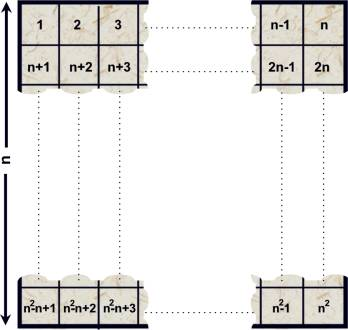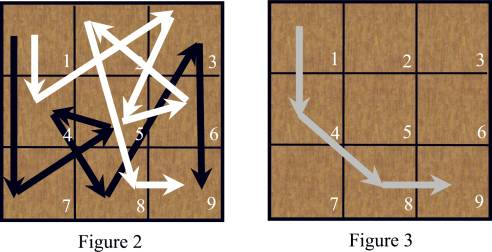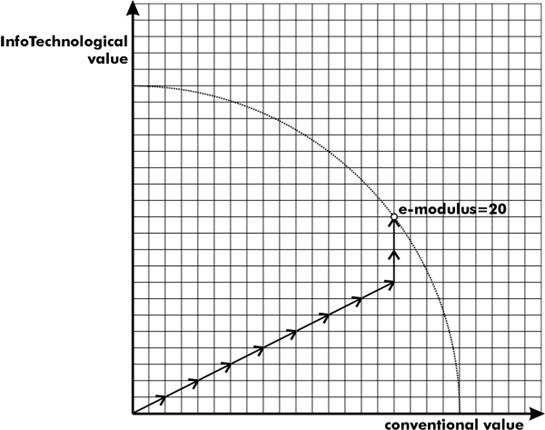## Background

$w=x_{1}x_{2}x_{3}\ldots{}x_{n}$

$$x_{i} \in \left\{ \begin{matrix} \left\{ \texttt{bcdfghjklmnpqrstvwxyz} \right\} & \text{if }\mathit{i}\text{ is odd}\\ \left\{ \texttt{aeiou} \right\} & \text{if }\mathit{i}\text{ is even} \end{matrix} \right.$$

$SBC(w) = \sum_{i\in{1\ldots{n}}} {i*P(x_{i})}$

a b c d e f g
12.53 1.42 4.68 5.86 13.68 0.69 1.01
h i j k l m n
0.70 6.25 0.44 0.00 4.97 3.15 6.71
o p q r s t u
8.68 2.51 0.88 6.87 7.98 4.63 3.93
v w x y z
0.90 0.02 0.22 0.90 0.52

## Sample Input

5
bubu
terabit
hacer
qed
loco


## Sample Output

below
above or equal
above or equal
above or equal
above or equal## Sample Input

1
3 6 7
1 7 5 4 8 3 9
1 4 3 5 6 2 8 9


## Sample Output

Case 1: 4$F(\theta{})=A\times{\sin{\theta}}+B\times{\cos{\theta}}$

1. 如果他不這麼做，新題目就會被生出來。: )
2. 在最近的比賽中，他放出來的題目被少於 10 隊的隊伍解出。
3. 他即將去一家世界有名的護目鏡生產公司。
4. 他在某天早上挖他家的後院時，挖到世足賽的門票。
6. 忘記了他和未婚妻的約會，跑去比同一天的 Topcoder SRM (Single Round Match)。(希望上帝能保佑他的靈魂！)
7. 舉辦一個程式競賽來慶祝他結婚。
8. 他快要訂婚了。
..................
99. 僅僅是因為他是優秀、善良又和藹的 Emoogle。


Emoogle Balance = Emoogle 說要請客的次數 - Emoogle 實際上請客的次數

Fiona 一直都很喜歡詩歌，最近她發現一個令人著迷的詩歌形式：重聲 (tautogram)，這是一種押頭韻 (alliteration) 的特殊情況，也就是說相鄰幾個單字的第一個字母都是一模一樣的。尤其是如果一個句子是重聲的話，那麼句子中所有單字的頭一個字母都是相同的。

• Flowers Flourish from France
• Sam Simmonds speaks softly
• Peter pIckEd pePPers
• truly tautograms triumph

Fiona 想讓他的男朋友看著滿是這些句子的情書看到頭昏眼花。請幫幫 Fiona 來確認每個句子是否是重聲。